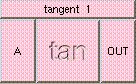TANGENTTHE BOX'S MAIN HELP: This function returns a scalar which runs to infinity as the angle A approachs PI/2, the tangent of angle A. A (input): This must be a scalar. This is the angle in degrees. OUT (output): This function returns a scalar which runs to infinity as the angle A approachs PI/2, the tangent of angle A.

<-- BACK TO Math